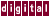## 4.2.3 /scheduling=<list>, /schd=<list>, (/scheduling=e)

The `/scheduling` qualifier tells KAP what kind of scheduling to use for the iterations of loops on a multiprocessor system. The possible values are as follows:

`e` = Even scheduling where KAP divides the iterations into equal size chunks so that there are at most (#_ of_processors) chunks. For example, if there are 9 iterations and 3 processors, the first processor would execute iterations 1, 2, and 3, the second processor, iterations 4, 5, and 6, and the third processor, iterations 7, 8, and 9.
`s` = Static scheduling where the processors execute iterations starting from their processor number and skipping (#_of_processors) iterations. For example, if there are 9 iterations and 3 processors, the first processor would execute iterations 1, 4, and 7, the second processor, iterations 2, 5, and 8, and the third processor, iterations 3, 6, and 9.

Previous Page Next Page Contents Index
Command-Line Qualifiers